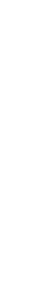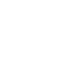To get a trial key
fill out the form below
* By clicking this button you agree to our Privacy Policy statementRequest our prices
--Select currency--
USD
EUR
RUB
* By clicking this button you agree to our Privacy Policy statementFree PVS-Studio license for Microsoft MVP specialists
* By clicking this button you agree to our Privacy Policy statementTo get the licence for your open-source project, please fill out this form
* By clicking this button you agree to our Privacy Policy statementI am interested to try it on the platforms:
 Windows Linux macOS PVS-Studio for .NET Core JetBrains Rider
* By clicking this button you agree to our Privacy Policy statementMessage submitted.

Your message has been sent. We will email you at

check your Spam/Junk folder and click the "Not Spam" button for our message.
This way, you won't miss messages from our team in the future.>
>
>
64-bit programs and floating-point calc…

# 64-bit programs and floating-point calculations

Aug 18 2010
Author:

A developer who is porting his Windows-application to the 64-bit platform sent a letter to our support service with a question about using floating-point calculations. By his permission we publish the answer to this question in the blog since this topic might be interesting for other developers as well.

## The text of the letter

I want to ask you one particular question concerning 32 -> 64 bits migration. I studied articles and materials on your site and was very astonished at the discrepancy between 32-bit and 64-bit code I had encountered.

The problem is the following one: I get different results when calculating floating-point expressions. Below is a code fragment that corresponds to this issue.

``````float fConst = 1.4318620f;
float fValue1 = 40.598053f * (1.f - 1.4318620f / 100.f);
float fValue2 = 40.598053f * (1.f - fConst / 100.f);``````

MSVC 32, SSE and SSE2 are disabled

/fp:precise: fValue1 = 40.016743, fValue2 = 40.016747

MSVC 64, SSE and SSE2 are disabled

/fp:precise: fValue1 = 40.016743, fValue2 = 40.016743

The problem is that the resulting values of fValue2 are different. Because of this discrepancy the code compiled for 32 bits and 64 bits produces different results what is invalid in my case (or perhaps invalid in any case).

Does your product detect anything related to this issue? Could you please tip me in what way 32/64 can impact the results of real arithmetic?

The Viva64 product does not detect such variations in a program's behavior after it's recompilation for the 64-bit system. Such changes cannot be called errors. Let's study this situation in detail.

## Simple explanation

Let's see first what the 32-bit compiler generates: fValue1 = 40.016743, fValue2 = 40.016747.

Be reminded that the float type has 7 significant digits. Proceeding from that we see that actually we get a value that is a bit larger than 40.01674 (7 significant digits). It does not matter if it is actually 40.016743 or 40.016747 because this subtle difference is out of the float type's accuracy limits.

When compiling in 64-bit mode, the compiler generates the same correct code whose result is the same "a bit larger than 40.01674" value. In this case, it is always 40.016743. But it does not matter. Within the limits of float type's accuracy we get the same result as in the 32-bit program.

So, once again the results of calculations on 32-bit and 64-bit systems are equal within the limitations of the float type.

## Stricter explanation

Accuracy of the float type is the value FLT_EPSILON that equals 0.0000001192092896.

If we add a value smaller than FLT_EPSILON to 1.0f, we will again get 1.0f. Only addition of a value equal to or larger than FLT_EPSILON to 1.0f will increase the value of the variable: 1.0f + FLT_EPSILON !=1.0f.

In our case, we handle not 1 but values 40.016743 and 40.016747. Let's take the largest of these two and multiple it by FLT_EPSILON. The result number will be the accuracy value for our calculations:

Epsilon = 40.016743*FLT_EPSILON = 40.016743*0.0000001192092896 = 0,0000047703675051357728

Let's see how much different numbers 40.016747 and 40.016743 are:

Delta = 40.016747 - 40.016743 = 0.000004

It turns out that the difference is smaller than the deviation value:

Delta < Epsilon

0.000004 < 0,00000477

Consequently, 40.016743 == 40.016747 within the limits of the float type.

## What to do?

Although everything is correct, unfortunately, it does not make you feel easier. If you want to make the system more deterministic, you may use the /fp:strict switch.

In this case the result will be the following:

MSVC x86:

/fp:strict: fValue1 = 40.016747, fValue2 = 40.016747

MSVC x86-64:

/fp:strict: fValue1 = 40.016743, fValue2 = 40.016743

The result is more stable but we still did not manage to get an identical behavior of 32-bit and 64-bit code. What to do? The only thing you can do is to put up with it and change the methodology of result comparison.

I do not know how much the following situation I want to describe corresponds to yours, but I suppose it is rather close.

Once I developed a computational modeling package. The task was to develop a system of regression tests. There is a set of projects whose results are looked through by physicists and estimated as correct. Code revisions brought into the project must not cause a change of output data. If pressure is at some moment t in some point is 5 atmospheres, the same pressure value must remain after adding a new button to the dialogue or optimizing the mechanism of initial filling of the area. If something changes, it means that there were revisions in the model and physicists must once again estimate all the changes. Of course it is supposed that such revisions of the model are quite rare. In normal development state of a project there must always be identical output data. However, it is in theory. In practice everything is more complicated. We could not get identical results every time even when working with one compiler with the same optimization switches. Results easily started to diffuse all the same. But since the project was even built with different compilers, the task of getting absolutely identical results was admitted as unsolvable. To be exact, perhaps the task could be solved but it would require a lot of efforts and lead to an inadmissible slow-down of calculations because of the impossibility to optimize the code. The solution appeared in the form of a special result comparison system. What is more, values in different points were compared not merely with the Epsilon accuracy but in a special way. I do not remember now all the specifics of its implementation but the idea was the following. If in some point processes run that make the maximum pressure of 10 atmospheres, the difference of 0.001 atmosphere in some other point is considered an error. But if a process is running in areas with pressure of 1000 atmospheres, the difference of 0.001 is considered an admissible error. Thus, we managed to build a rather secure system of regression testing that, as I believe, has been working successfully to this day.

## The last thing: why do we get different results in 32-bit and 64-bit code at all?

It seems that the reason lies in using different sets of instructions. In 64-bit mode, these are SSE2 instructions which are always used nowadays and which are implemented in all the processors of the AMD64 (Intel 64) family. By the way, because of this, the phrase in your question "MSVC 64, SSE and SSE2 are disabled" is incorrect. SSE2 are used by the 64-bit compiler anyway.

## References

Popular related articles

Next comments